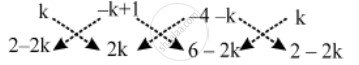# For What Value of k Are the Points (k, 2 – 2k), (–k + 1, 2k) and (–4 – k, 6 – 2k) Are Collinear ? - Mathematics

Sum

For what value of k are the points (k, 2 – 2k), (–k + 1, 2k) and (–4 – k, 6 – 2k) are collinear ?

#### Solution

Given points will be collinear, if area of the triangle formed by them is zero.

We have,i.e.,

|{2k^2 + (–k + 1) (6 – 2k) + (–4 – k) (2 – 2k)} – {(–k + 1) (2 – 2k) + (–4 – k) (2k) + k (6 – 2k)}| = 0

⇒ |(2k^2 + 6 –8k + 2k^2 + k^2 + 6k – 8) – (2 – 4k + k^2 – 8k – 2k^2 + 6k – 2k^2 )| = 0

⇒ (6k^2 – 2k – 2) – (–2k^2 – 6k + 2) = 0

⇒ 8k^2 + 4k – 4 = 0

⇒ 2k^2 + k – 1 = 0 ⇒ (2k – 1) (k + 1) = 0

⇒ k = 1/2 or, k = – 1

Hence, the given points are collinear for

⇒ k = 1/2 or, k = – 1.

Concept: Area of a Triangle
Is there an error in this question or solution?
Share